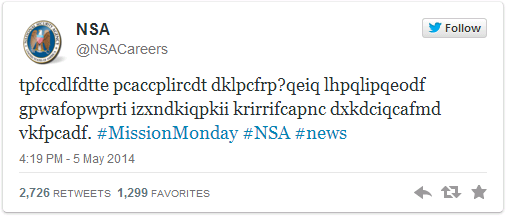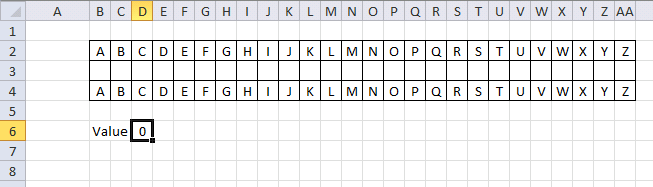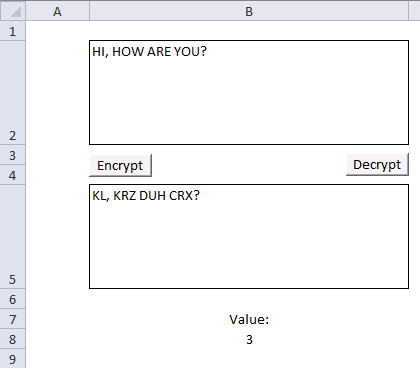Author: Oscar Cronquist Article last updated on May 20, 2014

A twitter account belonging to NSA posted this weird message one morning in the beginning of May.It is a basic substitution cipher meaning t=w, p=a and so on. The message is "Want to know what it takes to work at NSA? Check back each Monday in May as we explore careers essential to protecting our nation"

A famous substitution cipher is the Caesar cipher, rotating each letter a number of places.According to wikipedia, the cipher was reasonably secure at the time because Caesar's enemies would have been illiterate. :-)

The following macro rotates each letter in cell B2 by a number found in cell B8.VBA Code

```Sub Encrypt()
Dim enc As String
Dim res As String

enc = Range("B2")
For i = 1 To Len(enc)
If Asc(UCase(Mid(enc, i, 1))) < 91 And Asc(UCase(Mid(enc, i, 1))) > 64 Then
If (Asc(UCase(Mid(enc, i, 1))) + Range("B8")) > 90 Then
res = res & Chr(65 + (Asc(UCase(Mid(enc, i, 1))) + Range("B8") - 90))
ElseIf (Asc(UCase(Mid(enc, i, 1))) + Range("B8")) < 65 Then
res = res & Chr(90 - (64 - (Asc(UCase(Mid(enc, i, 1))) + Range("B8"))))
Else
res = res & Chr(Asc(UCase(Mid(enc, i, 1))) + Range("B8"))
End If
Else
res = res & Mid(enc, i, 1)
End If
Next i

Range("B5") = res

End Sub

Sub Decrypt()

Dim dec As String
Dim res As String
dec = Range("B5")
For i = 1 To Len(dec)
If Asc(UCase(Mid(dec, i, 1))) < 91 And Asc(UCase(Mid(dec, i, 1))) > 64 Then
If (Asc(UCase(Mid(dec, i, 1))) - Range("B8")) > 90 Then
res = res & Chr(65 + (Asc(UCase(Mid(dec, i, 1))) - Range("B8") - 91))
ElseIf (Asc(UCase(Mid(dec, i, 1))) - Range("B8")) < 65 Then
res = res & Chr(90 - (64 - (Asc(UCase(Mid(dec, i, 1))) - Range("B8"))))
Else
res = res & Chr(Asc(UCase(Mid(dec, i, 1))) - Range("B8"))
End If
Else
res = res & Mid(dec, i, 1)
End If
Next i

Range("B2") = res

End Sub
```